[数据库] SQL语句select简单记录总结数据库 同时被 2 个专栏收录23 篇文章 10 订阅

一. 创建数据库和表

1.创建数据库
--创建数据库
create database StudentMS

--使用数据库
use StudentMS

--删除数据库
--drop database StudentMS

2.创建表并设置主键（外键类似）
--创建学生表 (属性:姓名、学号(pk)、学院、出生日期、性别、籍贯)
create table xs
(
name varchar(10) not null,
id varchar(10) not null,
xy varchar(10),
birthday datetime,
xb char(2),
jg varchar(8)
)

--创建学生表主键:学号
alter table xs
pk_xs primary key(id)

--创建表学生表外键:系代号 此表中xdh已被省略
alter table xs
fk_xs foreign key(xdh)
references xb (xdh)

3.插入数据
insert into xs
(id, name, xb, birthday, xy, jg)
values('1160001', '刘备', '男', '1991-11-5', '软件学院', '河北省');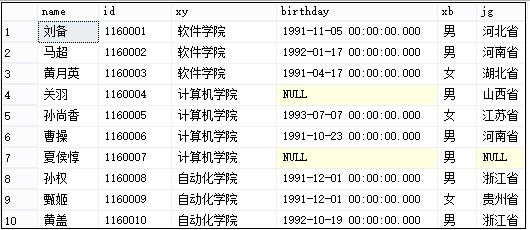二. select查询操作

1.通过日期计算年龄

1). year(getdate()) - year(birthday)
2). datediff(YY, birthday, getdate())

select id as 学号, name as 姓名, year(getdate())-year(birthday) as 年龄, birthday as 出生日期
from xs;

select id as 学号, name as 姓名, datediff(YY,birthday,getdate()) as 年龄, birthday as 出生日期
from xs;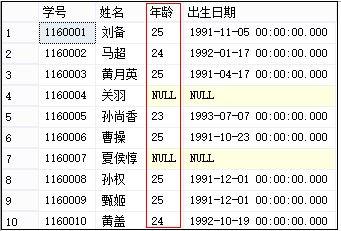Trunc(MONTHS_BETWEEN(SYSDATE, BIRTH_DATE)/12)
函数Trunc在这里对带有小数位数的数字取整数部分，SYSDATE为oracle的获取当前日期的函数，BIRTH_DATE为我自己的数据库表中存储生日日期的字段。
判断年份等于当前年份方法：YEAR=to_char(sysdate, 'yyyy')
如果BIRTH_DATE是字符串类型，则计算年龄语句如下：
Trunc(MONTHS_BETWEEN(SYSDATE, to_date(BIRTH_DATE,'yyyy-mm-dd'))/12)

2.获取某列所有不同的属性值 group by

--方法1：group by 列分组
select xy from xs group by xy;

--方法2：列出不同的值
select distinct xy from xs;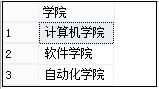select xy as 学院, count(*) as 总人数 from xs group by xy;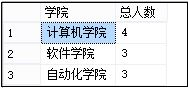PS：如果需要排序可以添加order by xy，而统计重名学生可通过having count(*)>1。

3.查询字符串匹配like和多值属性判断in
LIKE 操作符用于在 WHERE 子句中搜索列中的指定模式：SQL LIKE 操作符 - w3school
--匹配姓名以"黄"开头的学生
select * from xs where name like '黄%';

--匹配学院包含"计算机"的学生
select * from xs where xy like '%计算机%';

--匹配姓名以"尚香"结尾的学生
select * from xs where name like '%尚香';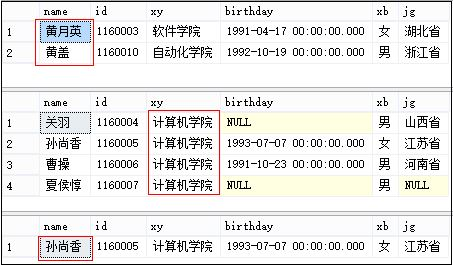LIKE匹配某个字段的变量的方法：DL_BHXNZYMC like '%' || ZY_NAME ||'%'
select T_WSTB_DLPYJBQKB.DL_BHXNZYMC, ZY_NAME
from T_WSTB_ZYJBQK, T_WSTB_DLPYJBQKB
where T_WSTB_DLPYJBQKB.DL_BHXNZYMC like '%' || ZY_NAME ||'%'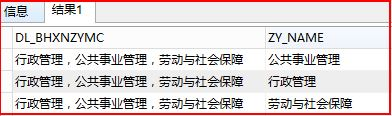IN 操作符允许我们在 WHERE 子句中规定多个值，换种说法就是替换or：
select * from xs where jg in ('河北省','河南省');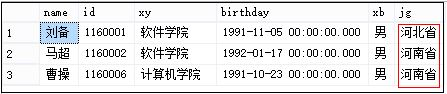4.查询输出某列属性中的某个特定值

select xy as 学院名称 from xs where xy='软件学院' group by xy;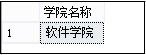5.子查询统计不同阶段学生总数

--子查询统计人数
select a.a_num as 软院人数, b.b_num as 计院人数, c.c_num as 自动化人数,
d.d_num as 男生人数, e.e_num as 女生人数, f.f_num as 河北河南人数
from
(select count(*) as a_num from xs where xy='软件学院') a,
(select count(*) as b_num from xs where xy='计算机学院') b,
(select count(*) as c_num from xs where xy='自动化学院') c,
(select count(*) as d_num from xs where xb='男') d,
(select count(*) as e_num from xs where xb='女') e,
(select count(*) as f_num from xs where jg in ('河北省','河南省')) f;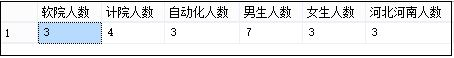PS：当时需要设计一条SQL，统计各个学院的教师总数、高级职称教师总数、35岁以下青年教师总数、教授教师总数；而且输出是一行，每个学院共5个值，例如：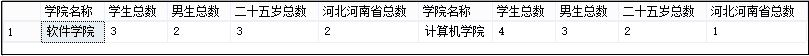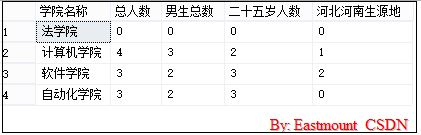6.使用子查询按行动态输出学院相关信息

--创建学院表
create table table_xy
(
name varchar(10) not null,
id varchar(10) not null
);

--插入数据
insert into table_xy(id, name) values('001', '软件学院');
insert into table_xy(id, name) values('002', '计算机学院');
insert into table_xy(id, name) values('003', '自动化学院');
insert into table_xy(id, name) values('004', '法学院');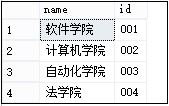select distinct name as 学院名称,
(select count(*) from xs where xs.xy=table_xy.name) as 总人数,
(select count(*) from xs where xs.xy=table_xy.name and xs.xb='男') as 男生总数,
(select count(*) from xs where xs.xy=table_xy.name and datediff(YY,birthday,getdate())<=25) as 二十五岁人数,
(select count(*) from xs where  xs.xy=table_xy.name and xs.jg in ('河北省','河南省'))　as 河北河南生源地
from table_xy;7.Oracle数据库null设置成自定义值的方法

1). nvl(expr1, expr2)

2). decode(DEPARTMENT_NAME, null, 'NULL', DEPARTMENT_NAME)

decode(person_name,null,“未知”, person_name)表示当person_name为空时返回“未知”，如不为空则返回person_name的字段值。

PS：而SQL Server中没有函数decode，但是其实质可以通过case when来实现和替代。

8.Oracle计算百分比trunc方法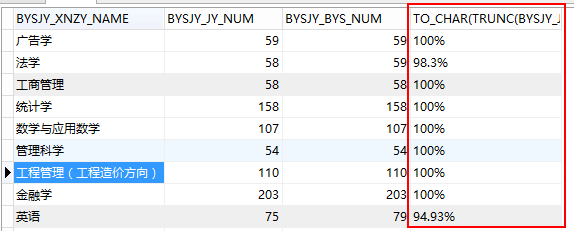9.Oracle查询除法/运算

[Err]ORA-01476: divisor is equal to zero，可修改为：select decode(b,0,0,a/b) from c。
select t1.ZFJGSL as 数量,
trunc( 1.0 * (select ITEM_VALUE from T_WSTB_YJBKBYSJYQK
where RECORD_YEAR=(to_char(sysdate, 'yyyy')-2) and FIRST_NUM='2' and SECOND_NUM='2') /
(select ITEM_VALUE from T_WSTB_YJBKBYSJYQK
where RECORD_YEAR=(to_char(sysdate, 'yyyy')-2) and FIRST_NUM='2' and SECOND_NUM='1') * 100,
2) as 比例
from
(select ITEM_VALUE as ZFJGSL from T_WSTB_YJBKBYSJYQK
where RECORD_YEAR=(to_char(sysdate, 'yyyy')-2) and FIRST_NUM='2' and SECOND_NUM='2') t1;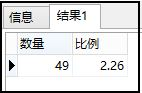10.Oracle统计某个属性逗号分隔值的个数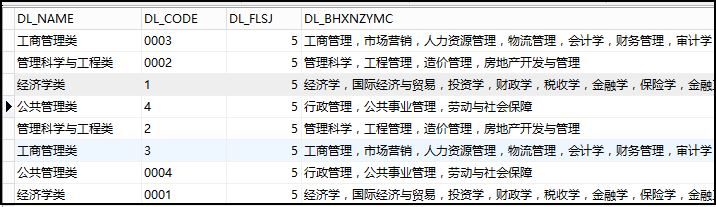SQL代码如下，Orcale使用length，其他是len函数：
select DL_NAME as num1,
length(DL_BHXNZYMC)-length(replace(DL_BHXNZYMC,'，',''))+1 as num2
from T_WSTB_DLPYJBQKB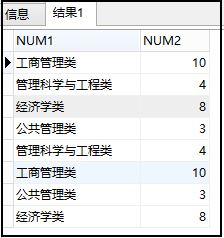11.Oracle使用CASE WHEN替代子查询

--子查询统计人数
select a.a_num as 软院人数, b.b_num as 计院人数, c.c_num as 自动化人数,
d.d_num as 男生人数, e.e_num as 女生人数, f.f_num as 河北河南人数
from
(select count(*) as a_num from xs where xy='软件学院') a,
(select count(*) as b_num from xs where xy='计算机学院') b,
(select count(*) as c_num from xs where xy='自动化学院') c,
(select count(*) as d_num from xs where xb='男') d,
(select count(*) as e_num from xs where xb='女') e,
(select count(*) as f_num from xs where jg in ('河北省','河南省')) f;SELECT COUNT(*) AS 总人数,
COUNT(CASE WHEN HIGHEST_DEGREE='博士'THEN 1 END) AS 博士人数,
COUNT(CASE WHEN HIGHEST_DEGREE='硕士'THEN 1 END) AS 硕士人数,
COUNT(*)-COUNT(CASE WHEN HIGHEST_DEGREE='博士'THEN 1 END)-COUNT(CASE WHEN HIGHEST_DEGREE='硕士'THEN 1 END) AS 其他学历
FROM T_WSTB_FULL_TIME_TEACHER
WHERE IS_FOREGOER='是' and YEAR_START=to_char(sysdate,'yyyy')-1;

COUNT(CASE WHEN HIGHEST_DEGREE='博士' THEN 1 END) AS NUM2

COUNT(CASE WHEN DW_NAME='软件学院' THEN NUM_STU END) AS NUM2

round((case when ZS!=0 then SHSJ/ZS else 0 end),3) as bl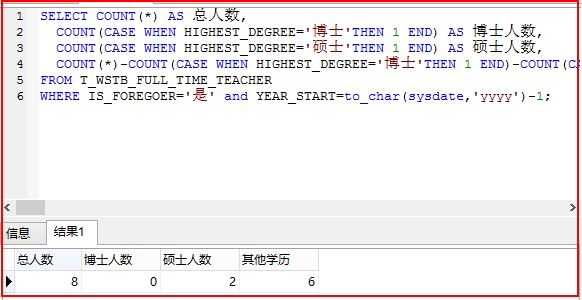12.Oracle子查询中使用a.* 统计所有字段

PS: 这部分代码是我的学生XW写的表2-6，非常不错，感觉自愧不如，学习之~
SELECT a.*, b.*
FROM
(SELECT SUM(DOMESTIC_TRAIN) + SUM(OVERSEA_TRAIN_TOTAL) AS zj,
SUM(DEGREE_PHD) AS qzgdbsx,
SUM(DOMESTIC_TRAIN) AS jnjxrcs,
SUM(OVERSEA_TRAIN_TOTAL) AS jwjxrcs
FROM TRAIN_INTERFLOW
where YEAR_START=to_char(sysdate,'yyyy')-2
) a,
(SELECT SUM(PARTICIPANT_NUMBER) AS cyjglxkrcs
FROM EDU_REVOLUTION
where YEAR_START=to_char(sysdate,'yyyy')-2
) b;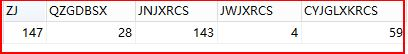13.Oracle使用decode函数防止除法分母为0
Oracle中通常需要统计如男生占全班总人数比例等用法，此时如果分母为0，它会报错
"[Err] ORA-01476: divisor is equal to zero"。那怎么办呢？

selecta/b from c; 修改成如下即可：select decode(b, 0, 0, a/b) from c;

select decode
(
(select sum(BYSJY_BYS_NUM) from T_WSTB_YJBKBYSFZYBYJYQK t
where t.BYSJY_XNZY_CODE=T_WSTB_ZYJBQK.XNZY_CODE),
0,
0,
(select sum(BYSJY_JY_NUM) from T_WSTB_YJBKBYSFZYBYJYQK t
where t.BYSJY_XNZY_CODE=T_WSTB_ZYJBQK.XNZY_CODE) * 1.0
/
(select sum(BYSJY_BYS_NUM) from T_WSTB_YJBKBYSFZYBYJYQK t
where t.BYSJY_XNZY_CODE=T_WSTB_ZYJBQK.XNZY_CODE)
) * 100 as JYL
from T_WSTB_ZYJBQK

round((case when ZS!=0 then SHSJ/ZS else 0 end),3) as bl

三. 总结

(By:Eastmount 2016-01-17 深夜3点   http://blog.csdn.net//eastmount/ )

07-075109
07-0273904-02228
07-281518
04-208503
01-1165
06-0411万+
03-203万+
04-0938
05-311123
06-13312
04-20342
02-143万+
03-172688
10-0987
02-073万+
11-05725
05-271126Eastmount

¥2 ¥4 ¥6 ¥10 ¥20余额支付 (余额：-- )扫码支付获取中扫码支付点击重新获取扫码支付1.余额是钱包充值的虚拟货币，按照1:1的比例进行支付金额的抵扣。
2.余额无法直接购买下载，可以购买VIP、C币套餐、付费专栏及课程。余额充值